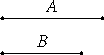# Proposition 7

Incommensurable magnitudes do not have to one another the ratio which a number has to a number.

Let A and B be incommensurable magnitudes.I say that A does not have to B the ratio which a number has to a number.

X.6

If A does have to B the ratio which a number has to a number, then A is commensurable with B.

But it is not, therefore A does not have to B the ratio which a number has to a number.

Therefore, incommensurable magnitudes do not have to one another the ratio which a number has to a number.

Q.E.D.

## Guide

This proposition is the contrapositive of the last one. It is used in X.11.